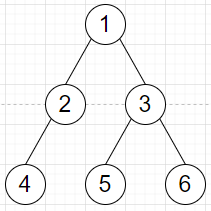# Check whether given degrees of vertices represent a Graph or Tree in Python

Suppose we have a list of degrees of some vertices. We have to check whether it is forming graph or tree.

So, if the input is like deg = [2,2,3,1,1,1], then the output will be TreeTo solve this, we will follow these steps −

• vert := number of vertices
• deg_sum := sum of all degree values of all vertices
• if 2*(vert-1) is same as deg_sum, then
• return 'Tree'
• return 'Graph'

Let us see the following implementation to get better understanding −

## Example Code

Live Demo

def solve(deg):
vert = len(deg)
deg_sum = sum(deg)

if 2*(vert-1) == deg_sum:
return 'Tree'
return 'Graph'

deg = [2,2,3,1,1,1]
print(solve(deg))

## Input

[2,2,3,1,1,1]


## Output

Tree

Updated on: 16-Jan-2021

97 Views